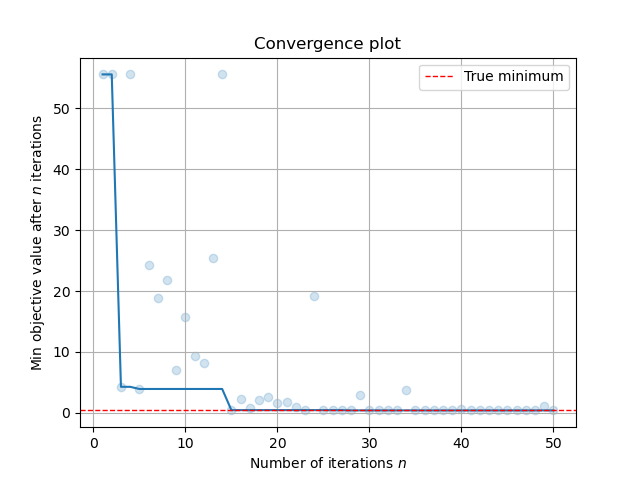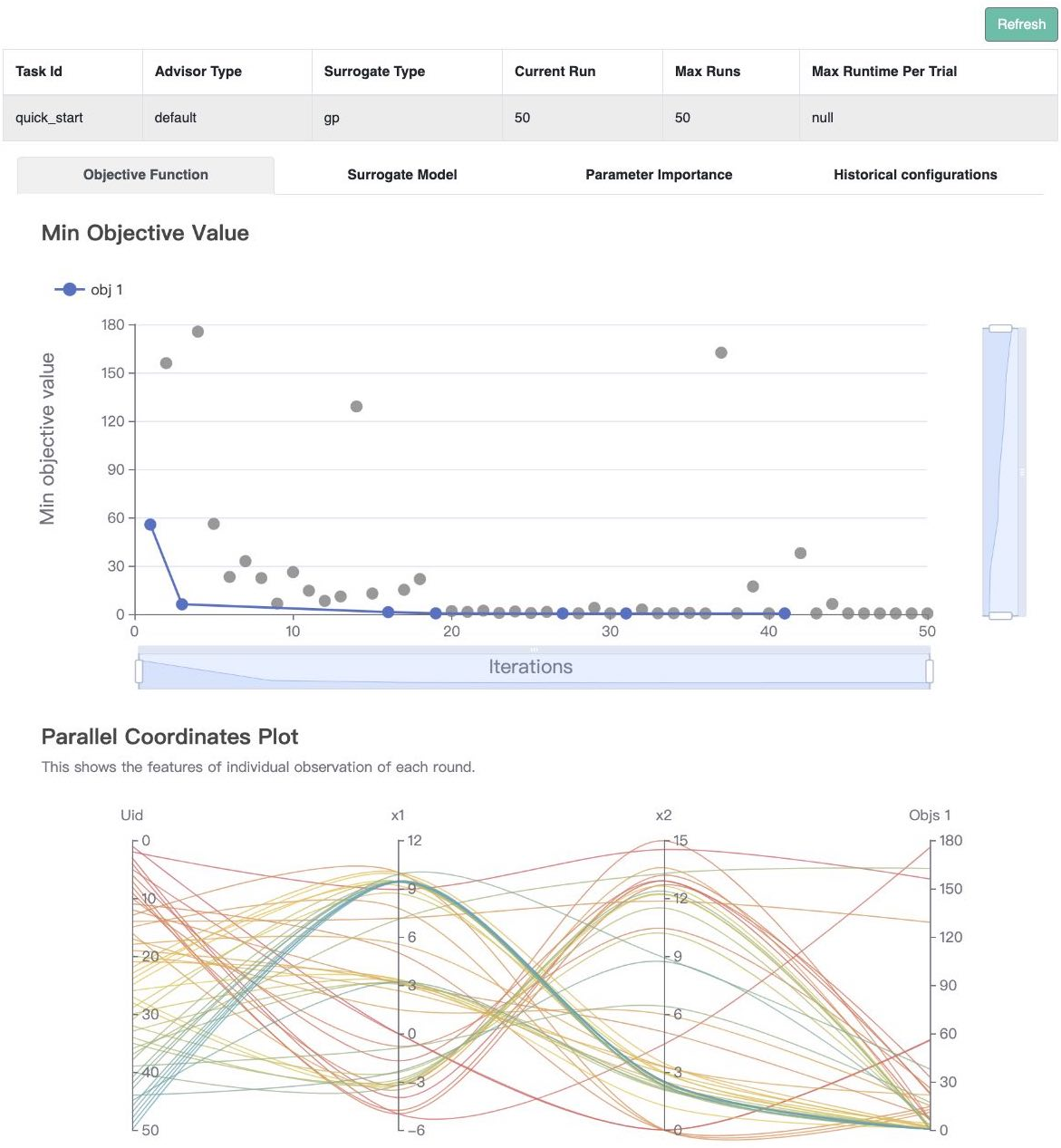# Quick Start#

This tutorial helps you run your first example with OpenBox.

## Space Definition#

First, define a search space.

from openbox import space as sp

# Define Search Space
space = sp.Space()
x1 = sp.Real("x1", -5, 10, default_value=0)
x2 = sp.Real("x2", 0, 15, default_value=0)


In this example, we create an empty search space, and then add two real (floating-point) variables into it. The first variable x1 ranges from -5 to 10, and the second one x2 ranges from 0 to 15.

OpenBox also supports other types of variables. Here are examples of how to define Int and Categorical variables:

from openbox import space as sp

i = sp.Int("i", 0, 100)
kernel = sp.Categorical("kernel", ["rbf", "poly", "sigmoid"], default_value="rbf")


For advanced usage, please refer to Problem Definition with Complex Search Space.

The space in OpenBox is implemented based on ConfigSpace package. For more usage, also refer to ConfigSpace’s documentation.

## Objective Definition#

Second, define the objective function to be optimized. Note that OpenBox aims to minimize the objective function. Here we provide an example of the Branin function.

import numpy as np

# Define Objective Function
def branin(config):
x1, x2 = config['x1'], config['x2']
y = (x2-5.1/(4*np.pi**2)*x1**2+5/np.pi*x1-6)**2+10*(1-1/(8*np.pi))*np.cos(x1)+10
return {'objectives': [y]}


The objective function takes as input a configuration sampled from space and outputs the objective value.

## Optimization#

After defining the search space and the objective function, we can run the optimization process as follows:

from openbox import Optimizer

# Run
opt = Optimizer(
branin,
space,
max_runs=50,
surrogate_type='gp',
# Have a try on the new HTML visualization feature!
# visualization='advanced',   # or 'basic'. For 'advanced', run 'pip install "openbox[extra]"' first
# auto_open_html=True,        # open the visualization page in your browser automatically
)
history = opt.run()


Here we create a Optimizer instance, and pass the objective function branin and the search space space to it. The other parameters are:

• num_objectives=1 and num_constraints=0 indicates our branin function returns a single value with no constraint.

• max_runs=50 means the optimization will take 50 rounds (optimizing the objective function 50 times).

• surrogate_type='gp'. For mathematical problems, we suggest using Gaussian Process ('gp') as Bayesian surrogate model. For practical problems such as hyperparameter optimization (HPO), we suggest using Random Forest ('prf').

• task_id is set to identify the optimization process.

• visualization: 'none', 'basic' or 'advanced'. See HTML Visualization.

• auto_open_html: whether to open the visualization page in your browser automatically. See HTML Visualization.

Then, opt.run() is called to start the optimization process.

## Visualization#

After the optimization, opt.run() returns the optimization history. Call print(history) to see the result:

print(history)

+-------------------------+-------------------+
| Parameters              | Optimal Value     |
+-------------------------+-------------------+
| x1                      | -3.138277         |
| x2                      | 12.254526         |
+-------------------------+-------------------+
| Optimal Objective Value | 0.398096578033325 |
+-------------------------+-------------------+
| Num Configs             | 50                |
+-------------------------+-------------------+


Call history.plot_convergence() to visualize the optimization process:

import matplotlib.pyplot as plt
history.plot_convergence(true_minimum=0.397887)
plt.show()Call print(history.get_importance()) to print the parameter importance: (Note that you need to install the pyrfr package to use this function. Pyrfr Installation Guide

print(history.get_importance())

+------------+------------+
| Parameters | Importance |
+------------+------------+
| x1         | 0.488244   |
| x2         | 0.327570   |
+------------+------------+


(New Feature!) Call history.visualize_html() to visualize the optimization process in an HTML page. For show_importance and verify_surrogate, run pip install "openbox[extra]" first. See HTML Visualization for more details.

history.visualize_html(open_html=True, show_importance=True,
verify_surrogate=True, optimizer=opt)